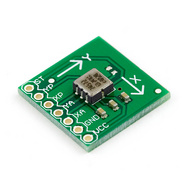# Getting an Angle

So probably the biggest part of constructing a balancing robot, or scooter, is knowing what angle it is in relation to the ground. From this, you can determine how much you need to compensate. The balancing system in my Segway consists of two sensors:*Above pictures from Sparkfun Electronics *

As I have explained in a previous post, both of these sensors are required to determine an accurate angle measurement. In my code so far, these are combined using a Complementary Filter. A great resource for coding a complementary filter on the Arduino can be found here. The hardest part that I found using this code is the Coefficients, which are not explained. I have determined, however, how to calculate these and get a working angle output. The 2 Coefficients in the program are 28.783 for the Gyro and 10.78 for the Accelerometer.

To calculate this for the Gyro:

1. Get the mv/°/s value for your Gyro from the Datasheet

-----25

1. Divide by 1000 (Milli volts in a volt)

-----0.025

1. Divide by 5 (Voltage of the Analog Reference)

-----.005

1. Divide by .0174532925 (Radians in a Degree)

-----0.2864789

1. Take the Inverse of that (1/x)

-----3.49

And there is your answer, 3.49. You would replace the 28.783 in the formula for this.

To calculate this for the Accelerometer:

1. Get the mv/g value for your Accelerometer from the Datasheet

-----1000

1. Divide by 1000 (Milli volts in a volt)

-----1

1. Divide by 5 (Voltage of the Analog Reference)

-----0.2

1. Take the Inverse

-----5

I'm not so sure on this one, but it seems to work. You would replace the 10.78 with this.

You can also replace the Voltage, in this case 5v, or the 1024 in the Formula, depending on your supply voltage or Analog Port Resolution.

When I put this all together in my test code, I get a solid, seemingly accurate angle measurement.

Next up - PID!